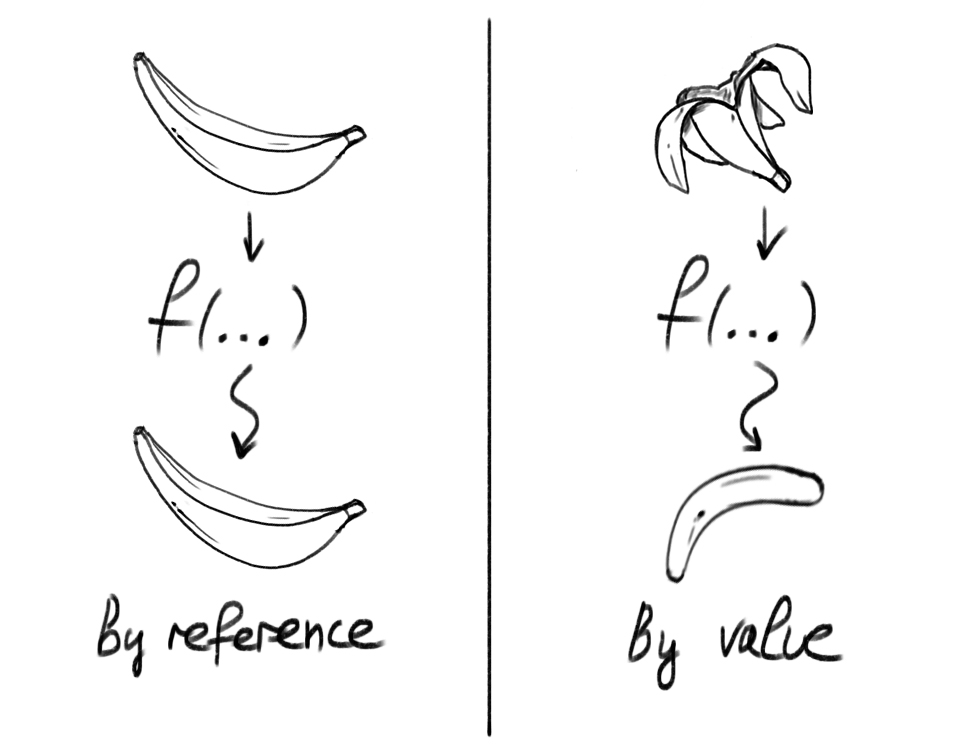# By reference or by value~ Basics ~In programming, there is the phrase "passing a variable". That is the moment when we call a function with a given parameter. In some languages, those parameters are passed by value in others by reference. In JavaScript we have a bit of a hybrid approach.

The primitives, like numbers and strings, are passed by value and some others like object literals and arrays are passed by "copy of a reference". Let's illustrate this with a couple of examples.

``````var numOfUsers = 24;
function doSomething(num) {
num += 1;
}

doSomething(numOfUsers);

console.log(numOfUsers); // 24
``````

Even though we define `numOfUsers` with `var`, we are passing it by its value. Meaning that inside the function, we receive its value, but we can not modify the original variable.

Here is another example:

``````const user = { score: 78 }
function mutates(obj) {
obj.score += 12;
}

mutates(user);
console.log(user.score); // 90
``````

Here `user` is passed by a copy of a reference. We can mutate its fields, but we can't amend the original definition. The following snippet proves that:

``````const user = { score: 78 }
function doesntMutate(obj) {
obj = { score: 120 }
}

doesntMutate(user);
console.log(user.score); // 78
``````

We have to make the note that in some other languages, the `obj = { score: 120 }` assignment will change the value of the `user` object.